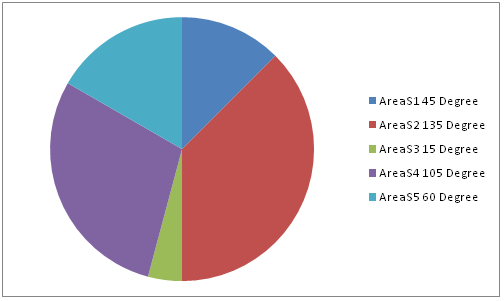# SSC CGL Exam: Numerical Ability: Concept & Sample Question: Data Interpretion

The SSC team of Jagranjosh.com has prepared topic wise study material for SSC CGL Exam.

Created On: Apr 7, 2015 15:21 IST
Modified On: Apr 8, 2015 12:20 ISTThe SSC team of Jagranjosh.com has prepared topic wise study material for SSC CGL Exam. The explanation of concept is done in way that every aspirant can easily understand the basics and tricks for the topic. The sample questions after concept study proves to be a milestone for understanding the concept very well.

Data Interpretation

Data

Data is defined as a rough collection of facts and figures which can be used to form a meaning information.

Data Interpretation

It is defined as the analysis of data given to find the various aspects like increase, decrease, average or changed in quantity of the article or thing which is given in data. Here, we are going to discuss the following five types of data interpretation

(1). Data table

Data Table

In data table, data is arranged systematically in rows and columns. We are only to read the information and data table carefully and answer the questions which are asked on it. There are generally two types of tables

There are generally two types of tables

(i) Simple table

(ii) Complicated table

Directions (Example) Read the table carefully and answer the questions that follow.Example 1. In 1994-95, the demand for flats is approximately what per cent of the total demand for steel?

(a) 26.4%

(b) 37.2%

(c) 35.6%

(d) 32.7%

Sol. (b) In 1994-95 demand for flats = 4360

Total demand = 6960 + 4360 +400 = 11720(2). Pie chart

Pie chart is called so because of its shape. Each slice of a pie is allowed to each category and shows the portion of the entire pie. In the questions of pie chart, the total quantity is distributed over a total angle of 360°..

Directions (Example) Refer to the following pie chart and answer the questions that follow.

National budget expenditure in the year 2000Example 1. In year 2000, if India had total expenditure of Rs 120 billion, how many billions did it spend on interest on debt?

(a) 10.8 billion

(b) 12.4 billion

(c) 14.2 billion

(d) 9.4 billion

Sol. (a) Total expenditure = 120 billion = 100%Bar Chart

A bar is a thick line whose width is shown merely for attention. In this method of data representation, the data is plotted on the x and y axes as bars.

Directions (Example) Study the following graph and answer the questions given below.Example 1. What was the percentage increase in production of salt in 2008 compared to that of 2001?

(a) 55.5

(b) 125

(c) 150

(d) 220Directions (Example 1-5) Read the given information carefully and answer the question given below. Population of five adjacent areas of a town, in the year of 2010, are represented in the following pie chart. The ratio of the numbers of males to that of females in these areas are stated in the table below. The total of the population in all the five areas is 72 lakh.Ratio of numbers of males (M) to females (F)

 Areas S1 S2 S3 S4 S5 Ratio M : F 3 : 2 4 : 1 7 : 3 2 : 3 13 : 7

Example 1. 12 lakh is the population of the area

(a) S1

(b) S3

(c) S5

(d) S4